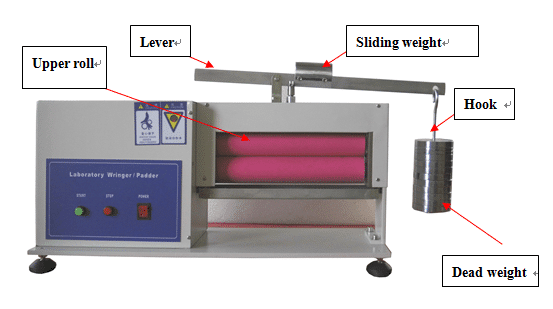+86-551-68 105003

The load between two rolls is supplied by the lever and dead weights.Mass 0.2kg 0.23kg 0.453kg
Quantity 1 piece 2 pieces 10 pieces

The ratio of the lever is 1:10.

The mass of lever is 1.5kg

The mass of upper roll is 3kg

The mass of sliding weight is 1kg

This instrument conforms to ISO 18696 Textiles-Determination of resistance to water absorption-Tumble-jar absorption test and AATCC TM70 Water Repellency: Tumble Jar Dynamic Absorption Test.

The load is required to be (27±0.5) kg in ISO 18696 and (27.2±0.5) kg in AATCC TM70 on fabric.

The load is applied in the following way.

• Position the sliding weight on the pivot;
• Hang the 2kg hook onto the level and then one piece of 0.23kg weight and one piece of 0.453kg weight;
• So the total weight (including the lever, the hook and the dead weights) is 2.383 (5kg+0.2kg+0.23kg+0.453kg);
• Because the ratio of lever is 1:10, the load supplied onto the upper roll is 83kg;
• The load supplied onto fabric is 83kg (23.83kg+3kg upper roll);

The load of 26.83kg is within (27±0.5) kg and (27.2±0.5) kg.

1.Denny says:
2.Andrea Chell says: